JAIC 1994, Volume 33, Number 2, Article 7 (pp. 153 to 170)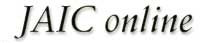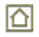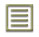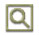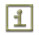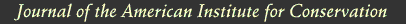JAIC 1994, Volume 33, Number 2, Article 7 (pp. 153 to 170)

### 3 STRESS DEVELOPMENT IN FULLY RESTRAINED MATERIAL RESULTING FROM CHANGES IN TEMPERATURE AND RELATIVE HUMIDITY

If artists' materials are fully restrained and subjected to decreases in either temperature or relative humidity, they will experience an increase in tension (Mecklenburg 1982; Hedley 1988; Berger and Russell 1986, 1988). This increase results from the materials' inability to contract while undergoing cooling or desiccation. The magnitude of the tensile stress the material experiences is a function of both the attempted shrinkage and the changing modulus of the materials.

In general, if the thermal coefficient of expansion and the equilibrium modulus of a material are known, the stress levels resulting from cooling a restrained material can be predicted. The method of calculating this stress increase can be derived directly from the basic eq 2, repeated here,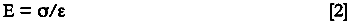which can be rewritten as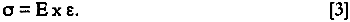When the modulus, E, and the strain, ∊, must be treated as functions rather than constants, eq 3 can be expressed as: for temperature,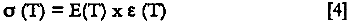and similarly for relative humidity,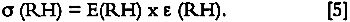Collecting the correct functions to program into the model therefore requires the determination of E(T), E(RH), ∊(T), and ∊(RH) for each of the individual components (i.e., paint, canvas, glue) in the object.

### 3.1 THE DETERMINATION OF STRAINS, ∊(T) AND ∊(RH), INDUCED BY TEMPERATURE OR RELATIVE HUMIDITY

The strain, ∊(T), as a function of temperature is derived by considering the shrinkage of the specimen as if it were free to do so. The specimen would contract upon cooling, and it is effectively being “stretched” back to its original restrained length. The “unstretched” length would be the free shrinkage length, and the deformation would be the amount needed to stretch the contracted specimen to the restrained length. The amount of shrinkage due to a temperature change is calculated from the thermal coefficient of expansion. Treating the thermal coefficient of expansion, γ, as a constant, the strain as a function of the temperature is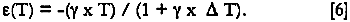The negative sign indicates that positive tensile strains are resulting from cooling. For changes in RH, the equation for the strains developed in a fully restrained material that is desicated are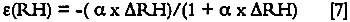where ΔRH is the change from the original RH to the new RH, with α the constant “moisture” coefficient of expansion. When coefficients of expansion do not hold constant over the environmental range of interest it becomes necessary to incorporate the moisture coefficient as a function of RH for increased accuracy in a numerical analysis.

### 3.2 SOME VALUES OF THERMAL AND MOISTURE COEFFICIENTS OF EXPANSION

The thermal coefficients of expansion for 13-year-old oil paints, hide glues, and photographic gelatins were measured. For one paint, a white lead, the thermal coefficient, γ, is 0.000052 per �C; for the rabbit skin glue, a thermal coefficient of about half that, or 0.000025 per �C, was measured. The thermal coefficient of expansion for photographic gelatin, γ, was 0.000030 per �C. These thermal coefficients were found to be reasonably constant over a measured range from 25�C to −10�C. In comparison, three common metals—copper, steel, and aluminum—have coefficients of 0.000017, 0.000011, and 0.000024 per �C respectively.

The swelling and shrinkage of oil paints with relative humidity is highly nonlinear but can be approximated as two linear sections from 10% to 70% RH and from 70% to 95% RH. The moisture coefficient of expansion can then be treated as a constant for each of the two respective humidity ranges. For example, the low RH range moisture coefficient, αa, for the 13-year-old-white lead is αa = 0.0000257/1%RH, which is the change in strain per 1% change in relative humidity. This coefficient was used to calculate the stresses resulting from the desiccation of restrained paint. For the high relative humidity range, αb = 0.000248/1% RH, which is nearly 10 times the low range coefficient. The coefficient for the high humidity range would be rarely used since the equilibrium modulus of paints above 70% RH is nil. A nonlinear treatment of the moisture coefficient is also possible. Rabbit skin glue, traditionally used in paintings, and gelatin, typically used in image emulsions and anticurl coatings on photographic products, are both quite dimensionally responsive to RH. This characteristic is not surprising because hide glues and gelatins are chemically very similar and can absorb high levels of water into their collagen structures. As with the paints, the low RH moisture coefficients are most useful. For the rabbit skin glue, αa is 0.000264/1%RH, more than 10 times that of the paints. For the more refined and processed photographic gelatin, αa is 0.00044/1%RH. Since most photographic emulsions are chemically “hardened” to some degree to reduce swelling and dissolution during photographic wet-processing procedures, highly hardened specimens cross-linked by formalin treatment were prepared in our laboratory and compared to unhardened specimens cast from the same gelatin. Interestingly, no significant difference in mechanical properties was found between unhardened and hardened gelatin. The fully developed nonlinear functions of the coefficient of expansion for photographic gelatin were as follows: between 15% and 60% RH,or, for even greater accuracy, between 12% and 85% RH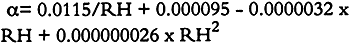where RH is the value of the current percent relative humidity. This equation is labeled “Best Fit” in figure 1.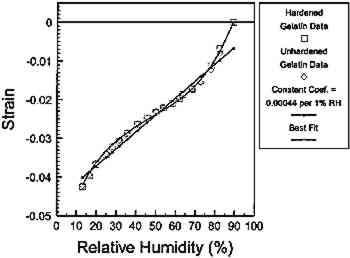### 3.3 THE DETERMINATION OF MODULUS, E(T) AND E(RH), INDUCED BY TEMPERATURE OR RELATIVE HUMIDITY

Several paints were mechanically tested under long-term loading conditions. Sample preparation and tests procedures are outlined elsewhere (Mecklenburg and Tumosa 1991b). The tests were conducted by applying a small strain to the test sample, about 0.007, and allowing it to stress relax fully. Once full stress relaxation was attained, a subsequent increment of strain was applied, and the stress was again allowed to relax. The time to fully relax the paints was typically between 5 and 10 days, depending on the paint. This process was repeated until the specimen broke. Each test took several months to complete. The modulus was determined by taking the slope of the locus of relaxed points from the equilibrium test (fig. 2). In this example for Naples yellow paint (lead white tinted with iron oxide in safflower oil) at 23�C, 50% RH, the modulus was 10,000 pounds per sq in (10 ksi), (68.9 Mega-pascals (MPa)). Similarly, the equilibrium modulus for the Naples yellow paint was measured at 23�C, 5% RH to be 47.5 ksi (327 MPa) and at −3�C, 5% RH was found to be 150 ksi (1,034 MPa). If it is assumed that E varies linearly with temperature, then a linear function for E(T) can be fitted and stated as: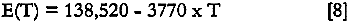where the units are psi for the modulus and the temperature is in degrees Celsius. The function was assumed to be linear since it was shown that the thermal coefficient was linear over the temperature ranges considered here.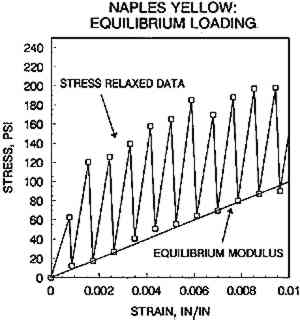For the Naples yellow paint, the complete equation for a fully restrained sample of paint subjected to a drop in temperature at 5% RH is now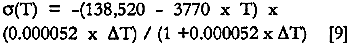where T is the current temperature (�C) and ΔT is the change from the original temperature to the current temperature.

From an experimental point of view, it is difficult to measure the stress of a fully restrained specimen since the load cell, the device that measures the stresses, is compliant. This means the cell gives a bit as force is applied to it. This load cell compliance effectively relieves some of the restraint on the specimen, and the stresses measured are actually less than if fully restrained. This compliance can, however, be included in the calculations so that the developed equations can be verified against the behavior of the specimens subjected to restraint and temperature changes. The compliance of the test device is a function of the total force and is measured during the test of the specimen. The value of the compliance, measured in units of length, is the raw data output, AO, times a compliance constant, KD. It is now possible to correct for the compliance of the load cell and calculate the expected stresses in the experimental restrained test where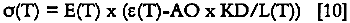where L(T) is the length of the specimen if allowed to shrink freely. The calculation of eq 9 was conducted for the Naples yellow paint using the measured modulus and the thermal coefficient of expansion of the material. The results of this calculation are presented in figure 3 as the lower continuous line. Also in that figure are the measured stress data (squares) for the Naples yellow paint, corrected for compliance of the test equipment as per eq 10. There is a substantial correlation between the predicted and the actual measured stress levels at the different temperatures while the RH is held at 5%. The temperature data were collected in the low 5% RH environment in order to isolate the effect of temperature from changes that would be caused by fluctuations in sample moisture content. Also shown in figure 3 are the test data for rabbit skin glue and a plotted line resulting from the determination of E(T) for the rabbit skin glue.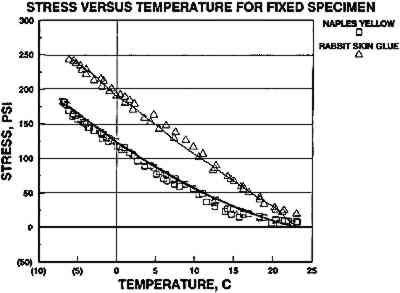E(RH) for Naples yellow paint was determined in the manner similar to that for restrained materials subjected to decreases in temperature discussed above. The results are shown in figure 4. One interesting aspect of these calculations is the remarkable accuracy using linear approximations of the actual material behavior. Equally interesting for the Naples yellow paint is the magnitude of stress reached, 0.066 ksi (0.455 MPa), when restrained and desiccated from 66% RH to 5% RH, which is considerably less than the stress, 0.145 ksi (1.0 MPa), reached when cooled from 23�C to −3�C at 5% RH.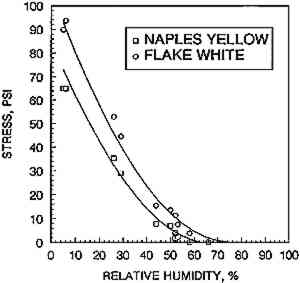### 3.4 THE EFFECTIVE MODULUS, Ee(RH), FOR RABBIT SKIN GLUE AND PHOTOGRAPHIC GELATIN

Until now all of the discussions about the mechanical properties of the materials and stress development in either cooled or desiccated environments have assumed that all behavior was linear. This assumption is valid since all of the elastic modulus equations result in successful stress predictions, as shown in figures 3 and 4. For the rabbit skin glue or gelatin subjected to changes in RH, the behavior is almost never in the elastic range but exhibits a quasi-plastic, nonlinear behavior. The reason for this behavior is that the material wants to shrink extensively with desiccation. This characteristic results in strains, when the specimen is restrained and desiccated, that exceed the yield point of the material. If the stress is eliminated the material will not return to its original length. The conventional definition of modulus, specified for the elastic regime of materials only, must be replaced by an “effective modulus” as a function of relative humidity, Ee(RH). This is done by rewriting eq 4 in the following form: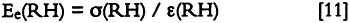where ∊(RH) is the strain determined from the previously measured moisture coefficient, as shown in eq 7, and σ(RH) is the measured stress taken from a restrained specimen test. Using a constant moisture coefficient of αa = 0.000264/1%RH, the effective modulus for rabbit skin glue is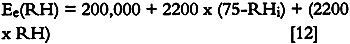where RHi is the value of the starting or initial relative humidity and RH is the relative humidity at any time.

For the photographic gelatin, using a constant moisture coefficient of 0.00044/1%RH, the effective modulus is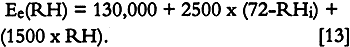The results of the glue and gelatin modulus functions are compared to actual data in figures 5 and 6. The sample specimens were restrained at different relative humidities and desiccated. Both of the materials showed similar behavior and reach similar maximum stress values at slightly over 4 ksi (27.6 MPa). This finding is of particular interest since the coefficient of expansion of the glue is lower than that of the gelatin, and the modulus of the glue is considerably higher than that of the gelatin.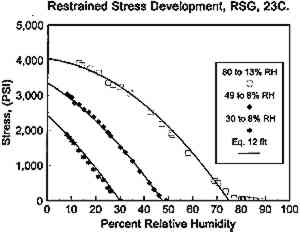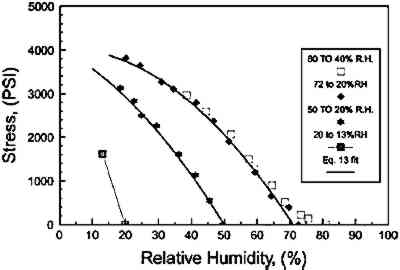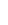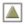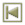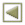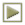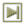Copyright � 1994 American Institute for Conservation of Historic and Artistic Works